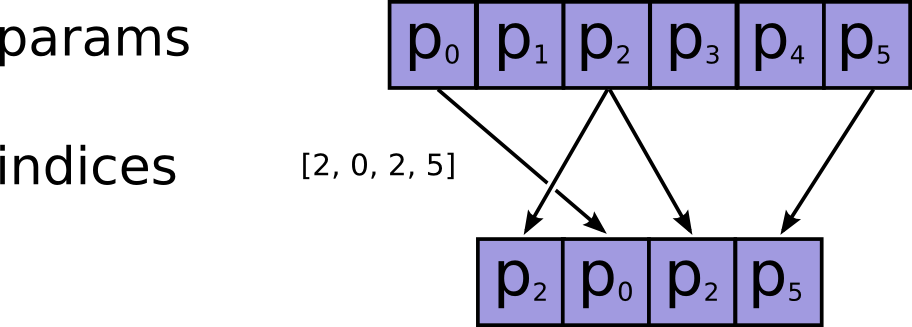Warning: This API is deprecated and will be removed in a future version of TensorFlow after the replacement is stable.

# Gather

public final class Gather

Gather slices from `params` axis `axis` according to `indices`.

`indices` must be an integer tensor of any dimension (usually 0-D or 1-D). Produces an output tensor with shape `params.shape[:axis] + indices.shape[batch_dims:] + params.shape[axis + 1:]` where:

``````# Scalar indices (output is rank(params) - 1).
output[a_0, ..., a_n, b_0, ..., b_n] =
params[a_0, ..., a_n, indices, b_0, ..., b_n]

# Vector indices (output is rank(params)).
output[a_0, ..., a_n, i, b_0, ..., b_n] =
params[a_0, ..., a_n, indices[i], b_0, ..., b_n]

# Higher rank indices (output is rank(params) + rank(indices) - 1).
output[a_0, ..., a_n, i, ..., j, b_0, ... b_n] =
params[a_0, ..., a_n, indices[i, ..., j], b_0, ..., b_n]
``````Note that on CPU, if an out of bound index is found, an error is returned. On GPU, if an out of bound index is found, a 0 is stored in the corresponding output value.

### Nested Classes

 class Gather.Options Optional attributes for `Gather`

### Public Methods

 Output asOutput() Returns the symbolic handle of a tensor. static Gather.Options batchDims(Long batchDims) static Gather create(Scope scope, Operand params, Operand indices, Operand axis, Options... options) Factory method to create a class wrapping a new Gather operation. Output output() Values from `params` gathered from indices given by `indices`, with shape `params.shape[:axis] + indices.shape + params.shape[axis + 1:]`.

## Public Methods

#### public Output<T> asOutput()

Returns the symbolic handle of a tensor.

Inputs to TensorFlow operations are outputs of another TensorFlow operation. This method is used to obtain a symbolic handle that represents the computation of the input.

#### public static Gather<T> create(Scope scope, Operand<T> params, Operand<U> indices, Operand<V> axis, Options... options)

Factory method to create a class wrapping a new Gather operation.

##### Parameters
scope current scope The tensor from which to gather values. Must be at least rank `axis + 1`. Index tensor. Must be in range `[0, params.shape[axis])`. The axis in `params` to gather `indices` from. Defaults to the first dimension. Supports negative indexes. carries optional attributes values
##### Returns
• a new instance of Gather

#### public Output<T> output()

Values from `params` gathered from indices given by `indices`, with shape `params.shape[:axis] + indices.shape + params.shape[axis + 1:]`.